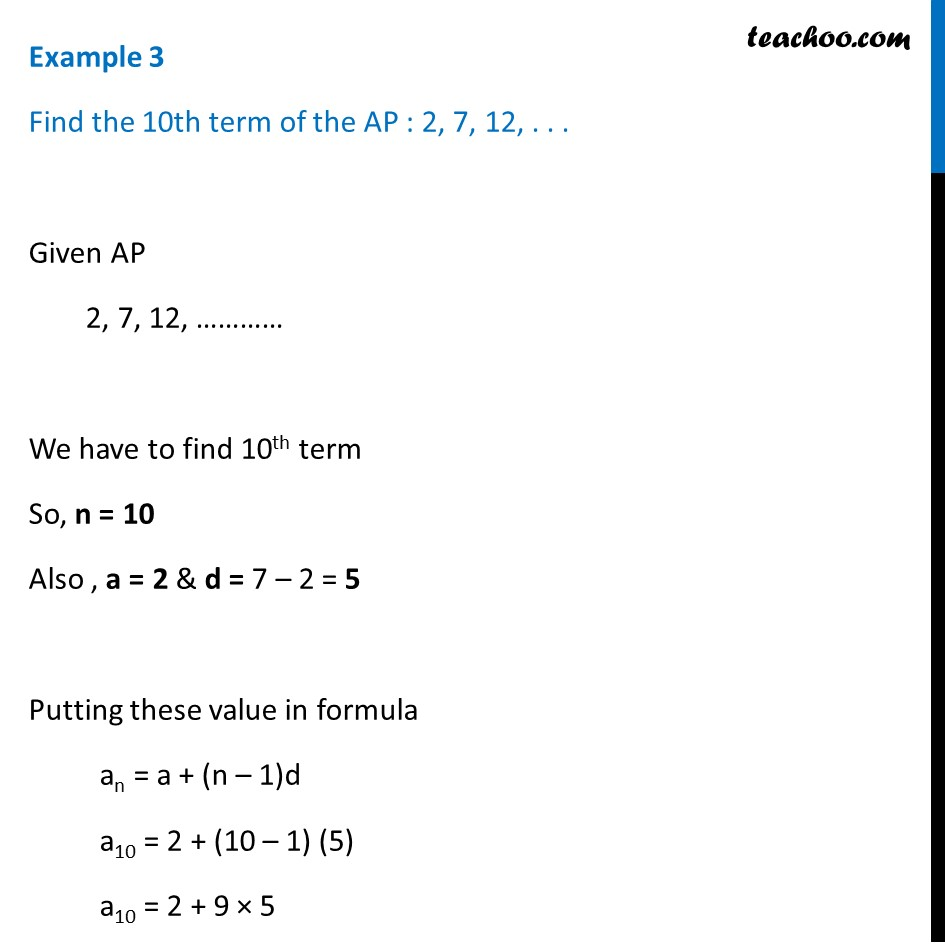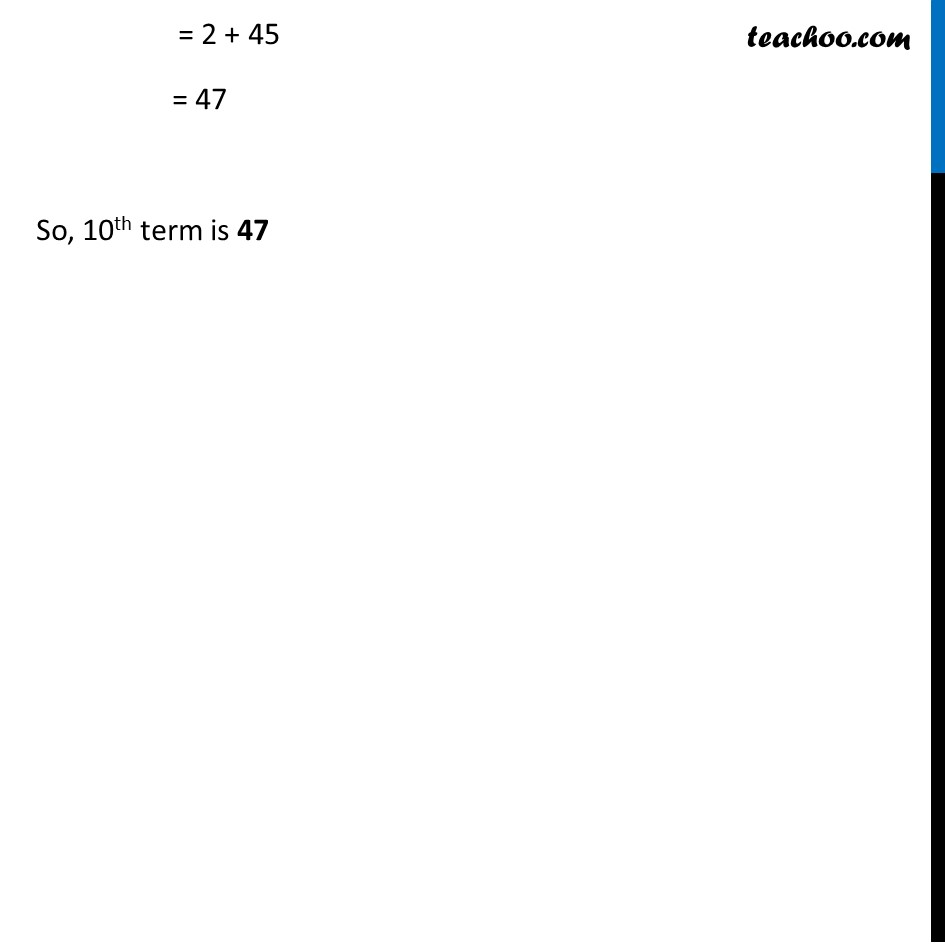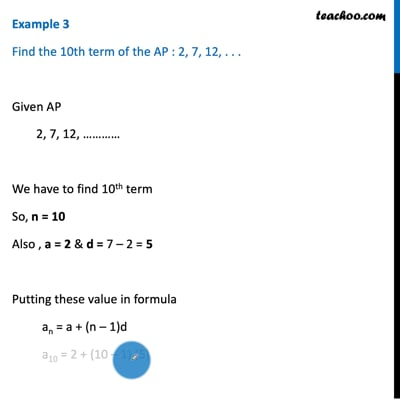Finding nth term

Chapter 5 Class 10 Arithmetic Progressions
Concept wiseThis video is only available for Teachoo black users

Introducing your new favourite teacher - Teachoo Black, at only ₹83 per month

### Transcript

Example 3 Find the 10th term of the AP : 2, 7, 12, . . . Given AP 2, 7, 12, ………… We have to find 10th term So, n = 10 Also , a = 2 & d = 7 – 2 = 5 Putting these value in formula an = a + (n – 1)d a10 = 2 + (10 – 1) (5) a10 = 2 + 9 × 5 = 2 + 45 = 47 So, 10th term is 47